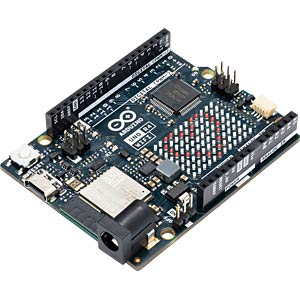I was wondering how to use the timers in the new Arduino UNO R4. Unfortunately I did not find any documentation, so I decided to document my findings here.The UNO R4 has two timer peripherals: the General PWM Timer (GPT) and the Asynchronous General Purpose Timer (AGT).

There are only 2 16 bit AGT timers (but one is already used to provide the Arduino millis() and microseconds() methods) and there are 7 GPT timers which are also used by PWM. To increase the flexibility you can explicitly request a timer which has been reserved for PWM.

The Arduino API implements the FspTimer class which provides all the necessary functionality.

Here is a test sketch that is setting up a GPT timer with a corresponding interrupt which executes a callback method at a defined interval rate e.g. 8000 hz.

``````#include "FspTimer.h"

FspTimer audio_timer;
uint64_t count=0;
uint64_t start_time=0;

// callback method used by timer
void timer_callback(timer_callback_args_t __attribute((unused)) *p_args) {
count++;
}

bool beginTimer(float rate) {
uint8_t timer_type = GPT_TIMER;
int8_t tindex = FspTimer::get_available_timer(timer_type);
if (tindex < 0){
tindex = FspTimer::get_available_timer(timer_type, true);
}
if (tindex < 0){
return false;
}

FspTimer::force_use_of_pwm_reserved_timer();

if(!audio_timer.begin(TIMER_MODE_PERIODIC, timer_type, tindex, rate, 0.0f, timer_callback)){
return false;
}

if (!audio_timer.setup_overflow_irq()){
return false;
}

if (!audio_timer.open()){
return false;
}

if (!audio_timer.start()){
return false;
}
return true;
}

void setup() {
Serial.begin(115200);
beginTimer(8000);
start_time = millis();
}

void loop() {
// calculate the effective frequency
int freq = 1000 * count / (millis()-start_time);
Serial.println(freq);
count = 0;
start_time = millis();
delay(1000);
}
``````

This is pretty straight forward!

The cool thing, which is no shown here, is that you can add a final parameter to the audio_timer.begin() method which will end up as parameter value which is passed to the timer_callback!

Categories: Arduino#### ArminJo · 26. July 2023 at 22:18

Hi Phil,
thanks for the code, but it is somehow incorrect, especially

if (tindex==0){

and

tindex = FspTimer::get_available_timer(timer_type);

at least for the 1.02 version of the FspTimer library

A correct version (with different variable names) should be:

```uint8_t tTimerType = GPT_TIMER; int8_t tIndex = FspTimer::get_available_timer(tTimerType); // Get first unused channel. Here we need the address of tTimerType if (tIndex < 0 || tTimerType != GPT_TIMER){ // here we found no unused GPT channel tIndex = FspTimer::get_available_timer(tTimerType, true); // true to force use of already used PWM channel // If we already get an tIndex < 0 we have an error, but do not know how to handle :-( FspTimer::force_use_of_pwm_reserved_timer(); // enable usage of channel for use in begin() } ```#### Doug · 3. July 2023 at 20:09

Thanks a lot for this posting. I just got an UNO r4, and quickly realized I had no idea how to implement a times ISR. Could find anything anywhere, and then saw this. Thanks again!!